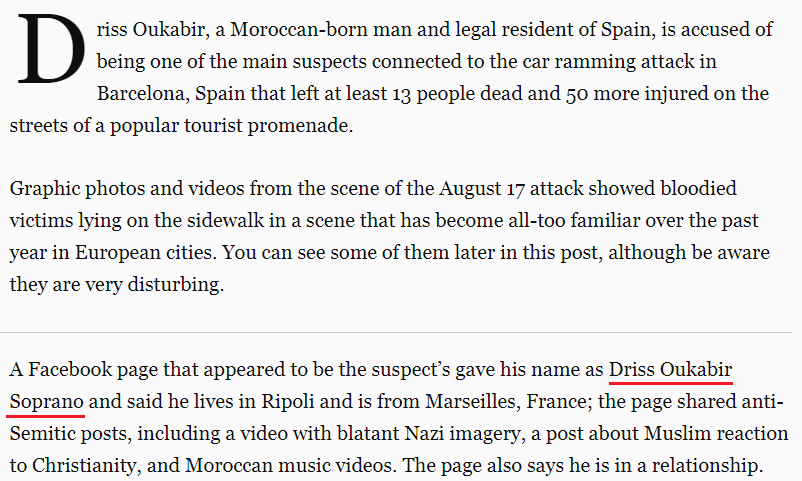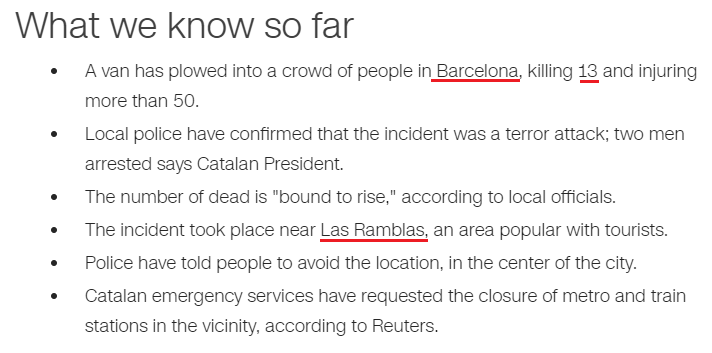## Cipher## Aug 18, 2017

### Deadly van attack in Spain, vehicle ramming attacks are trending

http://www.cnn.com/2017/08/17/europe/barcelona-spain-van-latest/index.html
This has become a monthly thing now...DEADLY VAN ATTACK IN SPAIN = 4+5+1+4+12+25 + 22+1+14 + 1+20+20+1+3+11 + 9+14 + 19+16+1+9+14 = 226 (English Ordinal)
August Seventeenth = 1+21+7+21+19+20 + 19+5+22+5+14+20+5+5+14+20+8 = 226(English Ordinal)
thirteen killed = 7+19+18+9+7+22+22+13 + 16+18+15+15+22+23 = 226 (Reverse Ordinal)
ISIS terror attack = 18+8+18+8 + 7+22+9+9+12+9 + 26+7+7+26+24+16 = 226(Reverse Ordinal)41 is the 13th prime number
Barcelona, Spain = 2+1+18+3+5+12+15+14+1 + 19+16+1+9+14 = 130 (English Ordinal)
This attack is happening in the sign of  "Leo" = 6+4+3 = 13 (Reverse Reduction)
"Deadly van attack" = 4+5+1+4+3+7 + 4+1+5 + 1+2+2+1+3+2 = 45 (Full Reduction)
Thirteen = 2+8+9+9+2+5+5+5 = 45 (Full Reduction)Suspect - Driss Oukabir Soprano = 4+9+9+1+1 + 6+3+2+1+2+9+9 + 1+6+7+9+1+5+6 = 91 (Full Reduction) - the 13th triangular number is 91
August Seventeenth = 26+6+20+6+8+7 + 8+22+5+22+13+7+22+22+13+7+19 = 233(Reverse Ordinal) - 233 is the 13th Fibonacci number and we see "At least thirteen dead" = 26+7 + 15+22+26+8+7 + 7+19+18+9+7+22+22+13 + 23+22+26+23 = 322 (Reverse Ordinal)"The incident took place near "Las Ramblas" = 15+26+8 + 9+26+14+25+15+26+8 = 172 (Reverse Ordinal)
Barcelona = 25+26+9+24+22+15+12+13+26 = 172 (Reverse Ordinal)Islamic State of Iraq and Syria = 9+1+3+1+4+9+3 + 1+2+1+2+5 + 6+6 + 9+9+1+8 + 1+5+4 + 1+7+9+9+1 = 117 (Full Reduction)
Central Intelligence Agency = 3+5+5+2+9+1+3 + 9+5+2+5+3+3+9+7+5+5+3+5 + 1+7+5+5+3+7 = 117 (Full Reduction)
CIA = 3+9+1 = 13 (English Ordinal)
Thirteen = 7+19+18+9+7+22+22+13 = 117 (Reverse Ordinal)Mike Pence = 4+9+2+5 + 7+5+5+3+5 = 45 (Full Reduction)
Thirteen = 2+8+9+9+2+5+5+5 = 45 (Full Reduction)
Mike Pence is giving a speech on 229th day of the year, exactly 9 months and 22 days before his next birthdayNote that his birthday is on 6/7 (6+7= 13)
6/7/1959 = 6+7+ 19 + 59 = 91 (the 13th triangular number)